Extrusion & Toll Manufacturing: 800-723-1792

Injection Molding, Tooling, & Mold Transfer: 413-443-1500

# Tensile Testing Results of Toner Plastics 3D Filament

• On November 23, 2016

# Mechanical Properties of Toner Plastics Filament

Written by: Robert Embury

# Summary

The experiments conducted were used to determine the material strength of five different types of 3D printer filaments. These materials consisted of PLA, ABS, ASA, PETG and Carbon Nano-Structure Composite ABS (CNS-ABS). The CNS-ABS material is currently in development and its additive ratios and therefore its mechanical properties are subject to change at any time. To find the material properties, a tensile test was performed on each of the specimens using an AccuView 1000 tensile machine. Using the data collected during the experiment, stress-strain diagrams were constructed for each specimen which allowed for the calculation and plotting of the following mechanical properties:

#### ABS

Young’s Modulus: Calculated: 116.4 KSI                    Injection Molded:334 KSI
Yield Stress: Calculated: 2891 PSI                            Injection Molded: 6520 PSI
Tensile Strength: Calculated: 5872 PSI                     Injection Molded: 5800 PSI
Elongation at Break: Calculated: 7.73%                    Injection Molded: 10.0%

CNS-ABS

Young’s Modulus: Calculated: 112.0 KSI                   Injection Molded: N/A
Yield Stress: Calculated: 2179 PSI                            Injection Molded: N/A
Tensile Strength: Calculated: 5755 PSI                     Injection Molded: N/A
Elongation at Break:Calculated: 6.88%                     Injection Molded: N/A

#### ASA

Young’s Modulus: Calculated: 117.0 KSI                     Injection Molded: 314 KSI
Yield Stress: Calculated: 2291 PSI                             Injection Molded: 7110 PSI
Tensile Strength: Calculated: 6381 PSI                      Injection Molded: N/A
Elongation at Break: Calculated: 8.30%                     Injection Molded: 20.0 %

#### PETG

Young’s Modulus: Calculated: 115.9 KSI                     Injection Molded: N/A
Yield Stress: Calculated: 2455 PSI                             Injection Molded: N/A
Tensile Strength: Calculated: 7079 PSI                      Injection Molded: N/A
Tensile Strength: Calculated: 9.16%                           Injection Molded: N/A

#### PLA

Young’s Modulus: Calculated: 143.0 KSI                      Injection Molded: 524 KSI
Yield Stress: Calculated: 2958 PSI                              Injection Molded: 8700 PSI
Tensile Strength: Calculated: 7964 PSI                       Injection Molded: 7690 PSI
Elongation at Break: Calculated: 7.31%                     Injection Molded: 6.0%

The data for the injection molded properties was taken from each material’s manufacturer data sheet. Each of the five materials tested failed due to shear failure. This is the expected method of failure for a ductile materials.

# Theory

The strength of a material is related to the material’s ability to resist deformation or failure while under a load. The relationship between stress and strain are useful because they provide insight into the material’s yield point, ultimate stress, elastic behavior and fracture stress. Using this data, it is possible to predict how a material will react when loaded. It can be used to determine if the material will return to its original dimensions after being deformed, when the material will begin yield and at what point the material will fail. Another useful piece of information gained from graphing stress-strain data is information on how different materials will fail. For example, a brittle material will fail due to cracking and separation while a ductile material will fail due to atomic plane slipping. The material properties and insight on the behavior of a material play a vital role in the selection process for engineering applications in which one or more specific properties may be required. The data collect from this experiment was taken in adherence to the ASTM D638 Tensile Test Standard on an AccuTest 1000 tensile machine. The graphs produced from tensile testing provide several key mechanical properties including:

Young’s Modulus (E): The slope of the stress-strain curve in the elastic region, where the deformation is reversible and time-independent. Basically, this is a measure of a material’s ability to resist permanent deformation.

Ultimate Strength (UTS): The maximum load sustained by the specimen divided by the original cross-sectional area of the specimen. This is the maximum stress the material can undergo before failure.

Yield Strength (σys): The stress on the stress-strain curve resulting in 0.2% permanent strain of the specimen based on its original length. This is the maximum stress a material can undergo before deforming permanently.

Percent Elongation at Break: The amount of deformation the specimen undergoes during testing as a percentage of its original length.

# Test Method

PLA, ABS, Carbon Nano-Structure Composite ABS, ASA and PETG samples were tested in adherence to the ASTM D638 Tensile Testing Standard for plastics. Each of the specimens was formed into a standard, flat, “dogbone” shape with a constant cross-sectional area. For the test, both sides of the specimen were lightly filed to roughen the surface to allow the jaws to properly grip the specimen.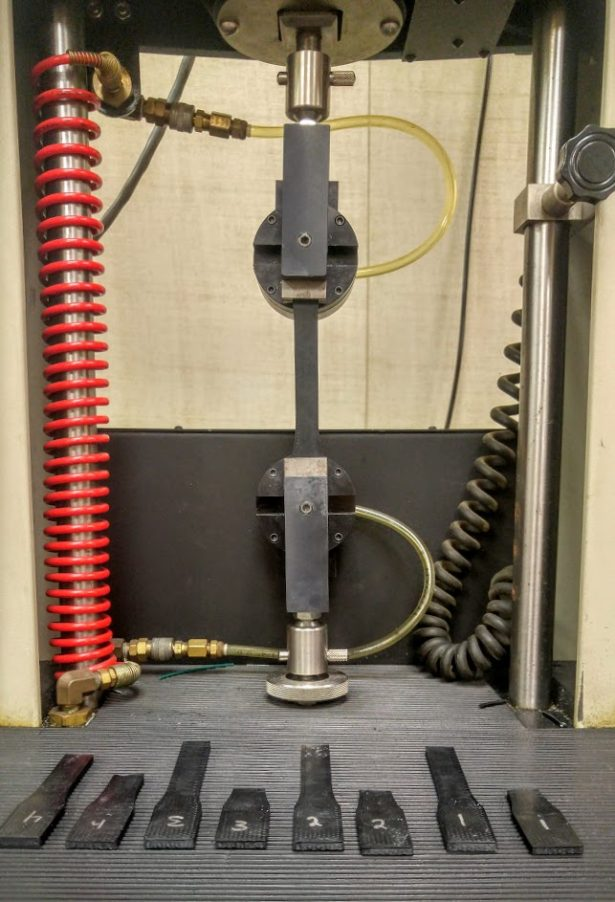Figure 1 – Tensile Testing Experimental Setup

Dimensions for the dogbone specimens can be seen below in Figure 2.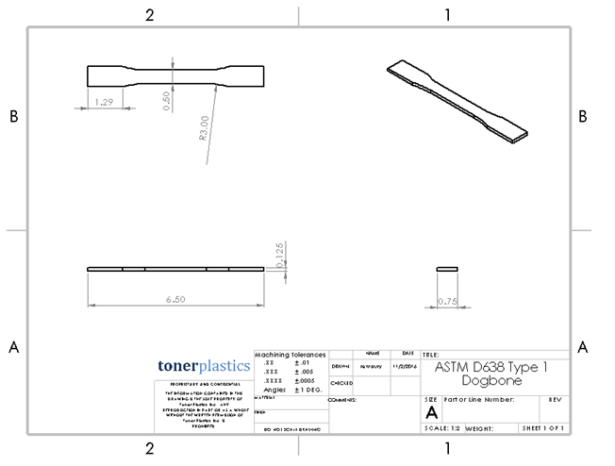Figure 2 – ASTM Dogbone Specimen Dimensions

For each material, 5 specimens were printed on a Lulzbot Taz 5 printer. The dogbones were printed lying flat on the build plate which would give the strongest part possible during tensile testing. An example of the specimen’s print orientation as well as the print settings for each can be found below in Figure 3 and in Table 1. Each part was allowed to cool for 24 hours before testing began. The data collected from the 5 tests of each material was averaged together to get a final value for each property.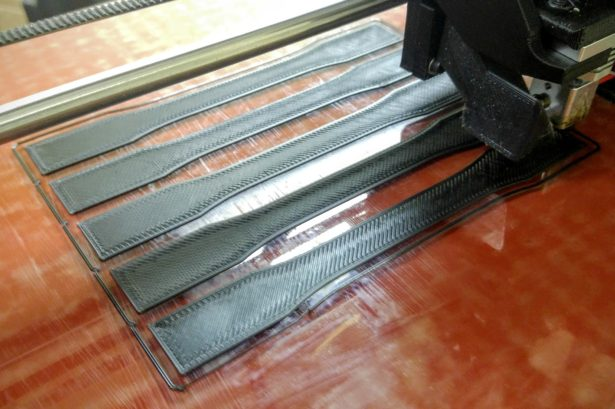Figure 3 – Test Specimen Print Orientation

Table 1 – Taz 5 Print Settings# Results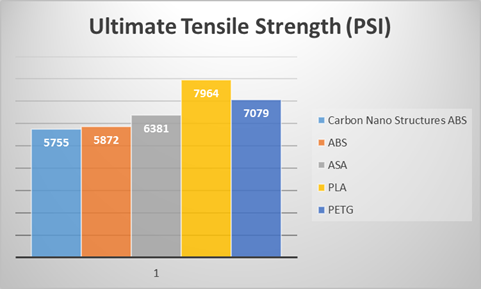Figure 4 – Ultimate Tensile Strength Results

Ultimate tensile strength is the maximum stress a material can withstand in tension before failing. The results for the ultimate tensile strength of each material matches up with its injection molded counterpart. PLA had the highest Uts at nearly 8000 PSI while CNS-ABS had the lowest at 5755 PSI.  It is interesting to note that the addition of carbon nano-structures in the CNS-ABS has only a small effect on its Uts in comparison to virgin ABS. ASA displayed an average ultimate tensile strength of 6381 PSI making it about 8% stronger in tension than ABS. PETG’s average ultimate tensile strength of 7079 PSI put it about 20% stronger than ABS.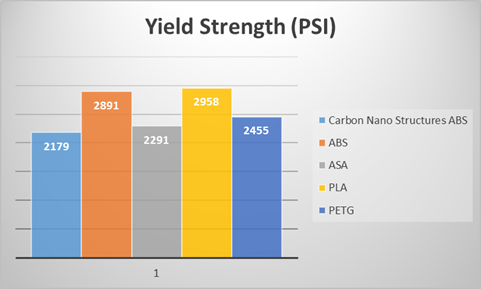Figure 5 – Yield Strength Results

Yield strength is the maximum stress a material can withstand in tension before permanent, plastic deformation occurs. ASA and CNS-ABS had a yield strength of 2291 PSI and 2179 PSI, respectively. Slightly above them was PETG with a yield strength of 2455 PSI. Both virgin ABS and PLA displayed a significantly higher yield strength over the other materials tested. PLA’s yield strength was 20% higher than PETG and 29% higher ASA.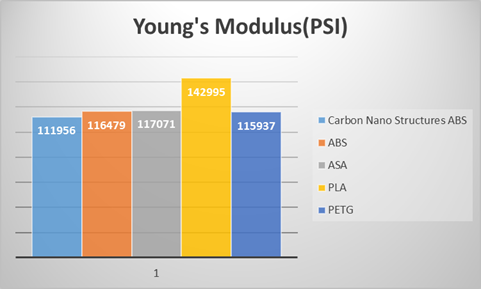Figure 6 – Modulus of Elasticity Results (Young’s Modulus)

Like the other test results, the Modulus of Elasticity of each material was positioned as expected in comparison to one another. PLA had the highest Young’s Modulus at about 143,000 PSI which represents a 73% reduction in strength when compared to its injection molded counterpart. All four other materials tested came in between 112,000 PSI and 117,000 PSI. For ABS and ASA, the Modulus of Elasticity of the 3D printed specimens where 65% and 62% less than an injection molded part.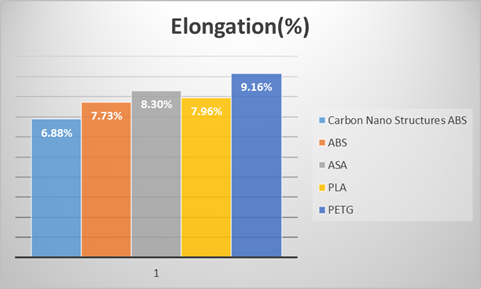Figure 7 – Elongation at Break

All five materials tested showed similar results with elongation at break.  As expected, the CNS-ABS had the lowest elongation at break which was approximately 11% less than that of virgin ABS. PLA has an average elongation at break of 7.96% putting it on par with ABS. On the other end of the scale, PETG displayed a 9.16% elongation at break representing a 30% increase over PLA while ASA had an 8.30% elongation at break.

# Conclusions

Several interesting pieces of information can be concluded from the results of this experiment:

• PLA can be a strong material choice for applications where resistance to permanent deformation is required or in applications where the printed part will be used in tension.
• If a part needs to bend rather than break, ABS and ASA are both good choices for the job with ASA providing a slightly higher ultimate tensile strength and maximum elongation.
• In addition to its UV resistance, ASA seems like the obvious choice over ABS.
• Overall, PETG proved to be a strong competitor with high tensile strength and maximum elongation and seems to be best suited for general purpose functional prints.
• CNS-ABS’ yield strength was significantly smaller than that of virgin ABS meaning that it took less force to cause the part to deform permanently.

One possible explanation of this is that the Carbon-Nano Structure additive had an adverse effect on the layer adhesion. Typically, injection molded parts with Carbon additives tend to display an increased tensile strength over their natural material counterparts.